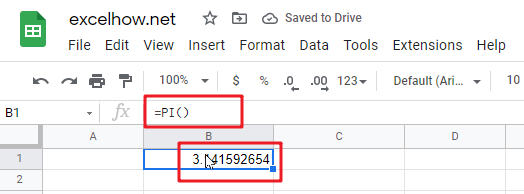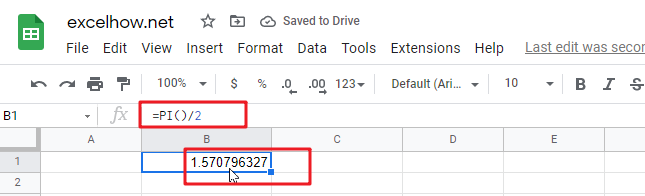This post will guide you how to use Google Sheets PI function with syntax and examples in Google Spreadsheets.

## Description

The Google Sheets PI function returns the value of mathematical constant PI. The returned value is 3.14159265358979 and ti will accurate to 15 digits.

The PI function is a build-in function in Google Spreadsheets and it is categorized as a Math and Trigonometry Function.

## Syntax

The syntax of the PI function is as below:

`=PI()`

The PI function has no any arguments.

## Google Sheets PI Function Examples

The below examples will show you how to use Google Sheets PI Function to return the value of PI.

1# get the value of PI, enter the following formula in Cell B1.

`=PI()`2# get the value of PI divided by 2, enter the following formula in Cell B2.

`=PI()/2`Related Posts

This post will guide you how to use Google Sheets MOD function with syntax and examples in Google Spreadsheets. Description The Google Sheets MOD function returns the remainder of two numbers after division. So you can use the MOD function ...

This post will teach you how to use Google Sheets COUNTIFS function with syntax and examples in Google Spreadsheets. Description The Google Sheets COUNTIFS function returns the count of cells in a range that meet one or more criteria. It ...